# Channel with multiple directions

(diff) ← Older revision | Latest revision (diff) | Newer revision → (diff)

multi-terminal channel, multi-user channel

A communication channel for which it is possible to transmit information in several directions simultaneously. The description which follows is for a memoryless discrete-time multi-user channel with finite input and output alphabets. Let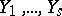befinite sets, where (the alphabet)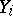is the set of possible signals which the-th transmitter can send, and letbefinite sets, where (the alphabet)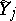is the set of possible signals which the-th receiver can receive. Suppose further that a stochastic matrix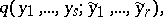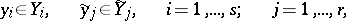is given. Two sets of random vectors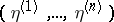and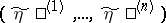, where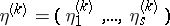,, defined on some probability space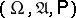, are said to be connected by a segment of lengthof a homogeneous multi-user channel withinputs andoutputs if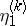and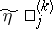, (;;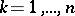), take values in the setsand, respectively, and the formula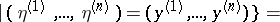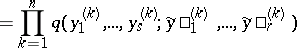holds for any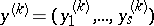and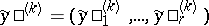,,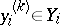,,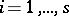;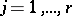.

The intuitive interpretation is that each input and each output of a multi-user channel is situated in a different terminal (end), i.e. there are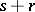terminals in total. This means that a transmitter or receiver situated in some terminal cannot use information known to the transmitters or the receivers of other terminals. Multi-user channels possessing this property are often called pure channels, in contrast to mixed multi-user channels, for which there exist terminals containing simultaneously several inputs and outputs of the channel. The complexity of the analysis of mixed multi-user channels is due to the fact that the transmitters of some terminal can, in selecting the next signal to be transmitted, use the information obtained at that moment by all the receivers of the given terminal; the receivers of this terminal can in turn use all the information available in the terminal at the given moment.

The most general problem in information transmission along a pure memoryless multi-user channel, as intuitively described above, is the following. Suppose that there are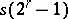discrete stationary sources of information(cf. Information, source of);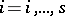,, whereis the set of all non-empty subsets of the index set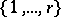generating the messages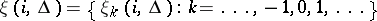, where the individual components of the message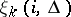take values in some set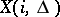of volume;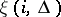can be required as the message intended for transmission from the-th input of the channel to all outputs with indices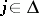. The message at the-th output,, is a set of random processes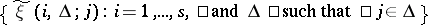, where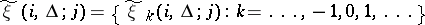and the components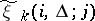take values in. Let segments of messages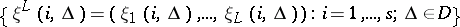of length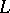be transmitted using a segment of lengthof a memoryless multi-user channel using the following block methods of (en)coding and decoding. The (en)coding is determined by a set of(en)coding mappingssuch that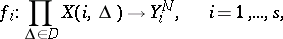(being the direct product of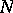copies of), and the decoding by a set of decoding mappings: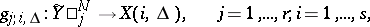andis such that.

The set of (en)coding functions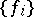establishes a functional dependence between the segments of lengthof the messages of all possible sources and the segments of lengthof the signals at the inputs of the channel. The set of decoding functions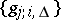establishes a functional dependence between the segments of lengthof the signals at the outputs of the channel and the segments of lengthof the messages generated at each output. If the joint distribution of the segments of lengthof the messages,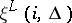, the (en)coding and decoding functionsand, and the transition probabilities of the memoryless multi-user channel are known, then one can calculate the error probability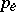, defined by the formula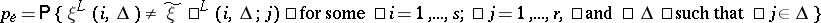.

A set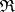of sets (vectors) of rates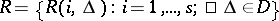in the-dimensional Euclidean space is called the capacity region of the channel considered here if for anythere exist an integer, (en)codingsand decodingssuch that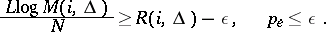The problem of describing the regionis one of the main problems in the theory of multi-user channels. In the general case this problem is unsolved. A final solution of it has been obtained only in certain special cases, for example, for multiple-access channels (that is, a multi-user channel with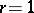) and for a certain class of broadcast channels (that is, channels with).

How to Cite This Entry:
Channel with multiple directions. Encyclopedia of Mathematics. URL: http://encyclopediaofmath.org/index.php?title=Channel_with_multiple_directions&oldid=13345
This article was adapted from an original article by R.L. DobrushinV.V. Prelov (originator), which appeared in Encyclopedia of Mathematics - ISBN 1402006098. See original article